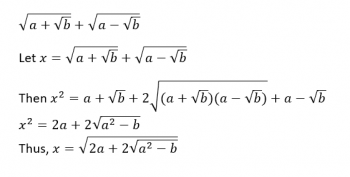Games
Problems
Go Pro!

"Sqr(2 + Sqr(3)) + Sqr(2 - Sqr(3)) works out to a simple radical (the square root of six). But not all radical expressions like that are so nice. How can you tell whether it'll simplify?" ~Paul

Hi Paul, whenever I have a question like this, I automatically think, "I'm going to replace the numbers with variables to see what happens."

So I'm going to rewrite your expression with variables, and then start manipulating it algebraically:So when does this expression not have a radical within a radical? Easy! When a2 - b is a perfect square! In your example, 22 - 3 = 1, which is a perfect square. By this reasoning, we could put any of the following ordered pairs (a,b) into the expression: (3, 5), (3, 8), (5, 21), (10, 19), and an infinite number of other possibilities!Like us on Facebook to get updates about new resources